# Texas Go Math Grade 5 Lesson 13.3 Answer Key Customary Capacity

Refer to our Texas Go Math Grade 5 Answer Key Pdf to score good marks in the exams. Test yourself by practicing the problems from Texas Go Math Grade 5 Lesson 13.3 Answer Key Customary Capacity.

## Texas Go Math Grade 5 Lesson 13.3 Answer Key Customary Capacity

Unlock the Problem

Mara has a can of paint with 3 cups of purple paint in it. She also has a bucket with a capacity of 26 fluid ounces. Will the bucket hold all of the paint Mara has?

The capacity of a container is the amount the container can hold.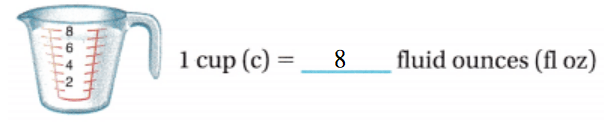A) What capacity does Mara need to convert?
The capacity that Mara needs to convert is: Fluid ounces (fl oz)

B)After Mara converts the units, what does she need to do next?
After Mara converts the units, she needs to find whether the bucket holds all of the paint Mara has.

Use a strip diagram to write an equation.

Step 1
Convert 3 cups to fluid ounces.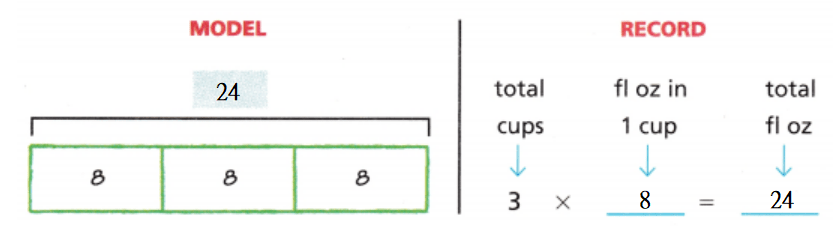Step 2Since 24 fluid ounces is less than 26 fluid ounces, Mara’s bucket _can not hold all of the paint.

• What if Mara has 7 cups of green paint and a container filled with 64 fluid ounces of yellow paint? Which color paint does Mara have more of? Explain your reasoning.
It is given that
Mara has 7 cups of green paint and a container filled with 64 fluid ounces of yellow paint
Now,
We know that,
1 cup = 8 fluid ounces
So,
The number of fluid ounces of green paint Mara has = 7 cups
= 7 × 8 fluid ounces
= 56 fluid ounces
So,
56 fluid ounces < 64 fluid ounces
Hence, from the above,
We can conclude that
Mara has more yellow paint

Example
Coral made 32 pints of fruit punch for a party. She needs to transport the punch in 1-gallon containers. How many containers does Coral need?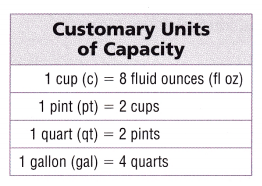To convert a smaller unit to a larger unit, you need to divide. Sometimes you may need to convert more than once.

Convert 32 pints to gallons.

Step 1:
Write an equation to convert pints to pints quarts.Step 2:
Write an equation to convert quarts to gallons.So,
Coral needs 256 1-gallon containers to transport the punch.

Share and Show

Question 1.
Use the picture to complete the statements and convert 3 quarts to pints.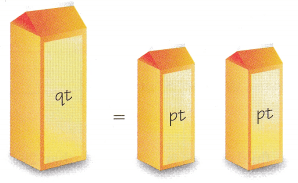The given figure is:Now,
From the given figure,
We can observe that
1 quart = 2 pints
So,
2 pints is greater than 1 pint
So,
a. 1 quart = 2 pints
b. 1 quart is greater than 1 pint.
c. 3 qt__ pt in 1 qt = ___ pt
We know that,
1 quart = 2 pints
So,
3 quarts = 3 × 2 pints
= 6 pints
Hence, from the above,
We can conclude that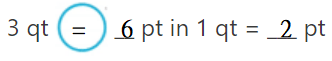Math Talk
Mathematical Processes
Explain how converting units of capacity is similar to converting units of length. How is it different?
In the metric system, the base unit of length is the meter (m). The base unit of capacity is the liter (L). The same prefixes are used throughout the metric system. The prefix of each unit of measurement indicates how that unit relates to the base unit

Convert.

Question 2.
3 gal = __ pt
The given unit is: Gallon
The converted unit must be: Pint
Now,
The given table is:Now,
From the above table,
We can observe that
1 gallon = 4 quarts
1 quart = 2 pints
So,
1 gallon = 4 × 2 pints
= 8 pints
So,
3 gallons = 3 × 8 pints
= 24 pints
Hence, from the above,
We can conclude that
3 gal = 24 pt

Question 3.
5 qt = __ pt
The given unit is: Quart
The converted unit must be: Pint
Now,
The given table is:Now,
From the above table,
We can observe that
1 quart = 2 pints
So,
5 quarts = 5 × 2 pints
= 10 pints
Hence, from the above,
We can conclude that
5 qt = 10 pt

Question 4.
6 qt = __ c
The given unit is: Quart
The converted unit must be: Cups
Now,
The given table is:Now,
From the above table,
We can observe that
1 quart = 2 pints
1 pint = 2 cups
So,
1 quart = 2 × 2 cups
= 4 cups
So,
6 quarts = 6 × 4 cups
= 24 cups
Hence, from the above,
We can conclude that
6 qt = 24 c

Problem Solving

Practice: Copy and Solve Compare. Write <, >, or =.

Question 5.
28 c14 pt
The given units are: Cups, Pints
Now,
The given table is:Now,
From the above table,
We can observe that
1 pint = 2 cups
So,
14 pints = 14 × 2 cups
= 28 cups
So,
28 c = 28 c
Hence, from the above,
We can conclude that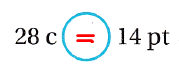Question 6.
25 pt13 qt
The given units are: Pints, Quarts
Now,
The given table is:Now,
From the above table,
We can observe that
1 quart = 2 pints
So,
13 quarts = 13 × 2 pints
= 26 pints
So,
25 pt < 26 pt
Hence, from the above,
We can conclude that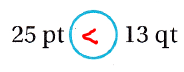Question 7.
20 qt80 c
The given units are: Quarts, Cups
Now,
The given table is:Now,
From the above table,
We can observe that
1 quart = 2 pints
1 pint = 2 cups
So,
1 quart = 2 × 2 cups
= 4 cups
So,
20 quarts = 20 × 4 cups
= 80 cups
So,
80 c = 80 c
Hence, from the above,
We can conclude thatQuestion 8.
Write Math Which of exercises 5-7 could you solve mentally? Explain your answer for one exercise.
We can solve Exercise 5 and Exercise 6 mentally
Now,
We know that,
1 pint = 2 cups
1 quart = 2 pints
Hence,
In Exercise 5,
14 pints = 14 × 2 cups
= 28 cups
= 28 c
Hence,
In Exercise 6,
13 quarts = 13 × 2 pints
= 26 pints
= 26 pt

Problem Solving

Show your work. For 9-11, use the table.

Question 9.
H.O.T. Use Graphs Complete the table and make a graph showing the relationship between quarts and pints.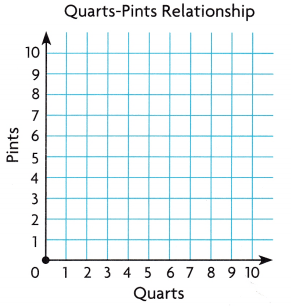The given table is: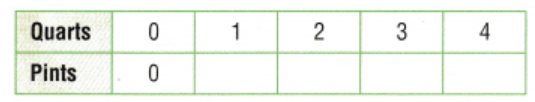Now,
We know that,
1 quart = 2 pints
So,
The completed table is:So,
The ordered pairs (x, y) are:
(0, 0), (1, 2), (3, 6), (4, 8)
Now,
The representation of the given ordered pairs in the coordinate plane is: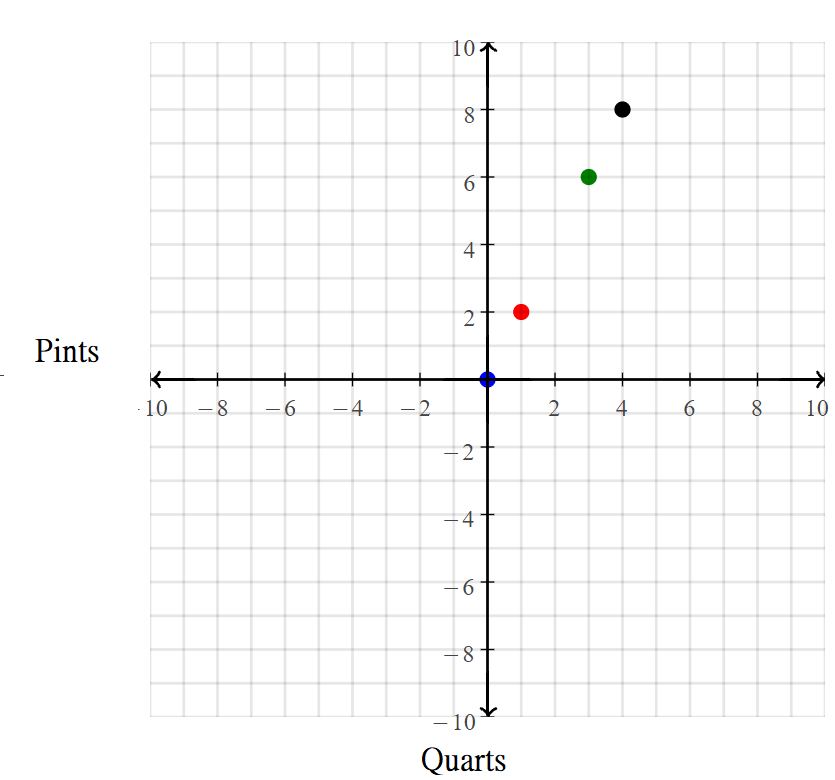Hence, from the above,
We can conclude that
The table and the graph that shows the complete relationship between the quarts and pints are:Question 10.
Multi-Step Describe any pattern you notice in the pairs of numbers you graphed. Write a rule to describe the pattern.
From Exercise 9,
We can observe that
The ordered pairs (x, y) for Quart – Pint relationship are:
(0, 0), (1, 2), (3, 6), (4, 8)
Now,
We know that,
1 quart = 2 pints
So,
The rule to describe the above pattern is:
The value of y is 2 times the value of x
Hence, from the above,
We can conclude that
The rule to describe the given pattern is:
The value of y is 2 times the value of x

Question 11.
What other pair of customary units of capacity have the same relationship as pints and quarts? Explain.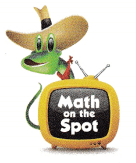The other pair of customary units of capacity that have the same relationship as pints and quarts are:
a. Cups
b. Gallons

Question 12.
Malcolm the Monster needs 3 quarts of fried ant eggs to make his favorite shampoo. How many pints of fried ant eggs does Malcolm need?
(A) 3 pints
(B) 1$$\frac{1}{2}$$ pints
(C) 6 pints
(D) 9$$\frac{1}{3}$$ pints
It is given that
Malcolm the Monster needs 3 quarts of fried ant eggs to make his favorite shampoo
Now,
We know that,
1 quart = 2 pints
So,
The number of pints of fried ant eggs does Malcolm need = 3 × 2 pints
= 6 pints
Hence, from the above,
We can conclude that
The number of pints of fried ant eggs does Malcolm need is: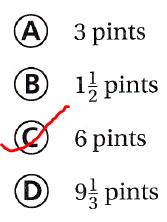Question 13.
Gina has a container that holds 32 fluid ounces of orange juice. What operation would she use to find how many gallons of orange juice the container holds?
(A) division
(C) multiplication
(D) subtraction
It is given that
Gina has a container that holds 32 fluid ounces of orange juice
Now,
The given table is:Now,
From the given table,
We can observe that
The “Gallon” is a larger unit when compared to “Fluid ounces”
Hence, from the above,
We can conclude that
The operation she would use to find how many gallons of orange juice the container holds is:Question 14.
Multi-Step Marco has 10 quarts of water and 20 cups of milk. How much liquid does he have in all?
(A) 60 cups
(B) 15 cups
(C) 60 quarts
(B) 15 pints
It is given that
Marco has 10 quarts of water and 20 cups of milk
Now,
The given table is:Now,
From the given table,
We can observe that
The amount of liquid does Marco have in all = 10 quarts + 20 cups
= (10 × 2 × 2) + 20    (or)     = (10 × 2) + 10 pints
= 60 cups                   (or)     = 30 pints
Hence, from the above,
We can conclude that
The amount of liquid does Marco have in all is: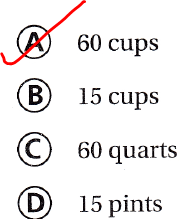Texas Test Prep

Question 15.
Shelby made 5 quarts of juice for a picnic. How many cups of juice did Shelby make?
(A) 1 cup
(B) 5 cups
(C) 10 cups
(D) 20 cups
It is given that
Shelby made 5 quarts of juice for a picnic
Now,
The given table is:Now,
From the given table,
We can observe that
1 quant = 2 × 2 cups
= 4 cups
So,
The number of cups of juice that Shelby made = 5 × 4 cups
= 20 cups
Hence, from the above,
We can conclude that
The number of cups of juice that Shelby made is:### Texas Go Math Grade 5 Lesson 13.3 Homework and Practice Answer Key

Compare. Write <, >, or =.

Question 1.
12c98fl oz
The given units are: Cups, Fluid ounces
Now,
The given table is:Now,
From the above table,
We can observe that
1 cup = 8 fluid ounces
So,
12 cups = 12 × 8 fluid ounces
= 96 fluid ounces
So,
96 fl oz < 98 fl oz
Hence, from the above,
We can conclude thatQuestion 2.
34 c18 pt
The given units are: Cups, Pints
Now,
The given table is:Now,
From the above table,
We can observe that
1 pint = 2 cups
So,
18 pints = 18 × 2 cups
= 36 cups
So,
34 c < 36 c
Hence, from the above,
We can conclude that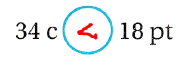Question 3.
16 pt8 qt
The given units are: Pints, Quarts
Now,
The given table is:Now,
From the above table,
We can observe that
1 quart = 2 pints
So,
8 quarts = 8 × 2 pints
= 16 pints
So,
16 pt = 16 pt
Hence, from the above,
We can conclude thatQuestion 4.
22 qt90 c
The given units are: Quarts, Cups
Now,
The given table is:Now,
From the above table,
We can observe that
1 quart = 2 pints
1 pint = 2 cups
So,
22 quarts = 22 × 2 pints × 2 cups
= 88 cups
So,
88 c < 90 c
Hence, from the above,
We can conclude that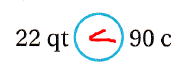Question 5.
19 pt9 qt
The given units are: Pints, Quarts
Now,
The given table is:Now,
From the above table,
We can observe that
1 quart = 2 pints
So,
9 quarts = 9 × 2 pints
= 18 pints
So,
19 pt > 18 pt
Hence, from the above,
We can conclude that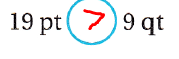Question 6.
20 gal75 qt
The given units are: Gallons, Quarts
Now,
The given table is:Now,
From the above table,
We can observe that
1 Gallon = 4 Quarts
So,
20 Gallons = 20 × 4 Quarts
= 80 Quarts
So,
80 qt > 75 qt
Hence, from the above,
We can conclude thatQuestion 7.
65 qt32 gal
The given units are: Quarts, Gallons
Now,
The given table is:Now,
From the above table,
We can observe that
1 Gallon = 4 Quarts
So,
32 Gallons = 32 × 4 Quarts
= 128 Quarts
So,
65 qt < 128 qt
Hence, from the above,
We can conclude thatQuestion 8.
28 c6 qt
The given units are: Cups, Quarts
Now,
The given table is:Now,
From the above table,
We can observe that
1 Quart = 2 pints
1 pint = 2 cups
So,
6 Quarts = 6 × 2 × 2 cups
= 24 cups
So,
28 c > 24 c
Hence, from the above,
We can conclude that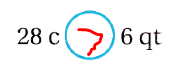Question 9.
15 gal120 pt
The given units are: Gallons, Pints
Now,
The given table is:Now,
From the above table,
We can observe that
1 Gallon = 4 Quarts
1 Quart = 2 pints
So,
15 Gallons = 15 × 4 × 2 pints
= 120 pints
So,
120 pt = 120 pt
Hence, from the above,
We can conclude that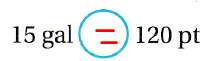Question 10.
Explain how you can solve exercise 3 mentally.
It is given that
In Exercise 3,
The given comparison is: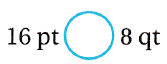Now,
We know that,
1 Quart = 2 pints
So,
8 Quarts = 8 × 2 pints
= 16 pints
Hence, from the above,
We can conclude that
By using the rule 1 Quart = 2 pints, we can solve Exercise 3 mentally

Problem Solving

Question 11.
Complete the table and make a graph showing the relationship between gallons and quarts.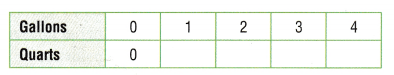The given table is:Now,
We know that,
1 Gallon = 4 Quarts
So,
The completed table is: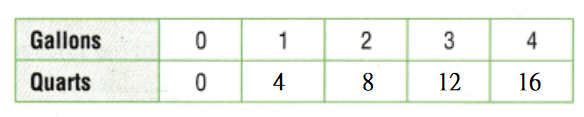So,
The ordered pairs (x, y) are:
(0, 0), (1, 4), (2, 8), (3, 12), (4, 16)
Now,
The representation of the given ordered pairs in the coordinate plane is: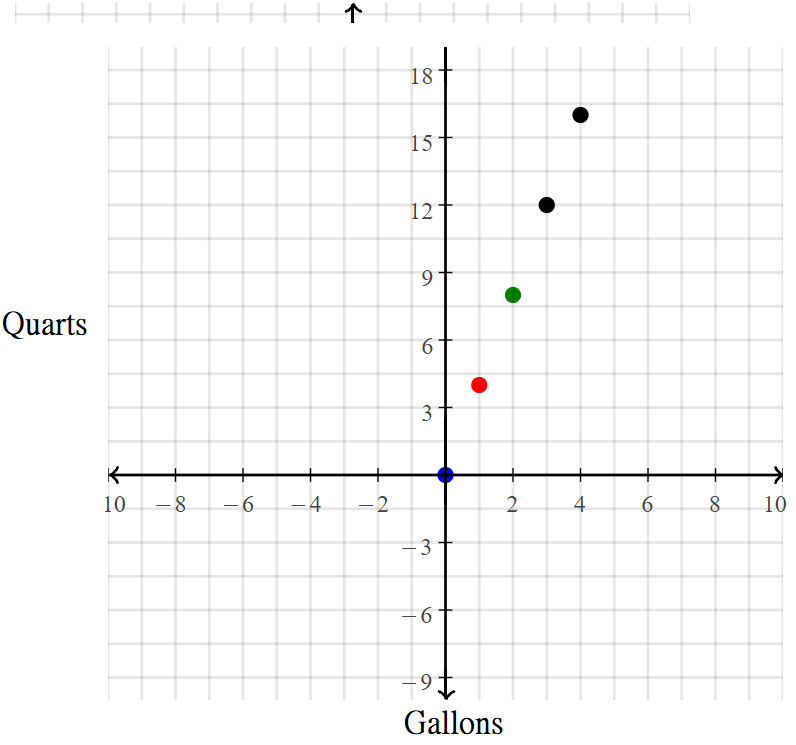Hence, from the above,
We can conclude that
The table and the graph that shows the complete relationship between the gallons and quarts are:Question 12.
Describe any pattern you notice in the pairs of numbers you graphed. Write a rule to describe the pattern.
From Exercise 11,
We can observe that
The ordered pairs (x, y) for Gallons- Quart relationship are:
(0, 0), (1, 4), (2, 8), (3, 12), (4, 16)
Now,
We know that,
1 Gallon = 4 Quarts
So,
The rule to describe the above pattern is:
The value of y is 4 times the value of x
Hence, from the above,
We can conclude that
The rule to describe the given pattern is:
The value of y is 4 times the value of x

Lesson Check

Question 13.
The frozen yogurt shop uses 1 pint of milk in each milkshake. How many milkshakes can be made with 24 quarts of milk?
(A) 96
(B) 12
(C) 6
(D) 48
It is given that
The frozen yogurt shop uses 1 pint of milk in each milkshake
Now,
The given table is:Now,
From the above table,
We can observe that
1 Quart = 2 pints
So,
The number of milkshakes can be made with 24 quarts of milk = 24 × 2
= 48 milkshakes
Hence, from the above,
We can conclude that
The number of milkshakes can be made with 24 quarts of milk is: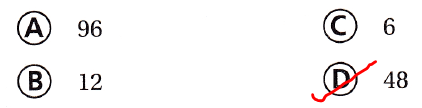Question 14.
What if the yogurt shop has 8 gallons of milk? How many milkshakes can be made from 8 gallons with 1 pint of milk in each shake?
(A) 64
(B) 32
(C) 128
(D) 16
It is given that
The yogurt shop has 8 gallons of milk
Now,
The given table is:Now,
From the given table,
We can observe that
1 gallon = 4 Quarts
1 Quart = 2 pints
So,
The number of milkshakes that can be made from 8 gallons with 1 pint of milk in each shake = 8 × 4 × 2
= 64 milkshakes
Hence, from the above,
We can conclude that
The number of milkshakes that can be made from 8 gallons with 1 pint of milk in each shake is:Question 15.
Allan needs 3 quarts of water to fill a pot for soup. How many times will he need to fill an 8-ounce measuring cup to fill the pot?
(A) 16
(B) 12
(C) 24
(D) 4
It is given that
Allan needs 3 quarts of water to fill a pot for soup
Now,
The given table is:Now,
From the above table,
We can observe that
1 Quart = 2 pints
1 pint = 2 cups
1 cup = 8 fluid ounces
So,
3 Quarts = 3 × 2 × 2 × 8
= 96 ounces
Now,
The number of times will Allan need to fill an 8-ounce measuring cup to fill the pot = $$\frac{96}{8}$$
= 12 times
Hence, from the above,
We can concldue that
The number of times will Allan need to fill an 8-ounce measuring cup to fill the pot is:Question 16.
Noriko and her friends made 26 pints of lemonade to sell at the fundraiser. Which operation will Noriko use to find how many cups of lemonade they have to sell?
(B) subtraction
(C) multiplication
(D) division
It is given that
Noriko and her friends made 26 pints of lemonade to sell at the fundraiser
Now,
The given table is:Now,
From the above table,
We can observe that
1 pint = 2 cups
Hence, from the above,
We can conclude that
The operation Noriko will use to find how many cups of lemonade they have to sell is:Question 17.
The paint store has 45 gallons of white paint in stock. How many quarts of white paint is this?
(A) 90 quarts
(B) 360 quarts
(C) 9 quarts
(D) 180 quarts
It is given that
The paint store has 45 gallons of white paint in stock
Now,
We know that,
1 Gallon = 4 Quarts
So,
The number of quarts of paint is 45 gallons of paint = 45 × 4 quarts
= 180 quarts
Hence, from the above,
We can conclude that
The number of quarts of paint is 45 gallons of paint is:Question 18.
Which expression can you use to convert 32 cups to pints?
(A) 32 ÷ 4
(B) 32 ÷ 2
(C) 32 × 2
(D) 32 × 4
The given table is:Now,
From the given table,
We can observe that
1 pint = 2 cups
So,
32 cups = 32 × $$\frac[1}{2}$$ pint
Hence, from the above,
We can conclude that
The expression that you used to convert 32 cups to pints is: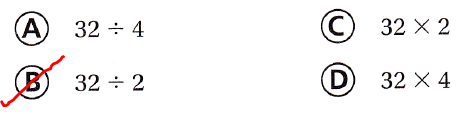Question 19.
Multi-Step The Holbrooks ordered 25 gallons of orange juice and 16 pints of cranberry juice for their restaurant. How much juice do they have in all?
(A) 57 gallons
(B) 29 gallons
(C) 216 pints
(D) 164 pints
It is given that
The Holbrooks ordered 25 gallons of orange juice and 16 pints of cranberry juice for their restaurant
Now,
The given table is:So,
The amount of total juice the Holbrooks have in all = (25 × 4 Quarts) + (16 × $$\frac{1}{2}$$ Quarts)
= 100 + 8
= 108 Quarts
= $$\frac{108}{4}$$ gallons
= 108 × 2
= 216 pints
= 27 gallons
Hence, from the above,
We can conclude that
The amount of total juice the Holbrooks have in all is: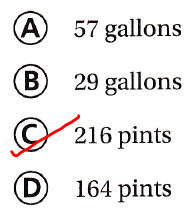Question 20.
Multi-Step Mrs. Sullivan calculates that she needs 12 gallons of paint to paint her house. She already has 8 quarts of the paint she needs. How many gallons of paint should she buy?
(A) 10 gallons
(B) 4 gallons
(C) 8 gallons
(D) 6 gallons
The amount of gallons of paint should Mrs. Sullivan buy = 12 gallons – $$\frac{8}{4}$$ gallons